# SSC Class 10 solutions for Maths Quadratic Equations Exercise 5.1,5.2,5.3 and 5.4

Mathematics class 10 SSC solutions for Quadratic equations are given.

Exercise 5.1, 5.2. 5.3 and 5.4 problems with solutions are given.

Observe the solutions and try them in your own method.

First you study the text book lesson Quadtratic Equations very well.

Observe the example problems and solutions goven in the textbook.

You can also see the text book solutions for

2. Sets

10. Mensuration

11. Trigonometry

13. Probability

14. Statistics

M

# Quadratic equations solutions class 10 ssc

Exercise 5.1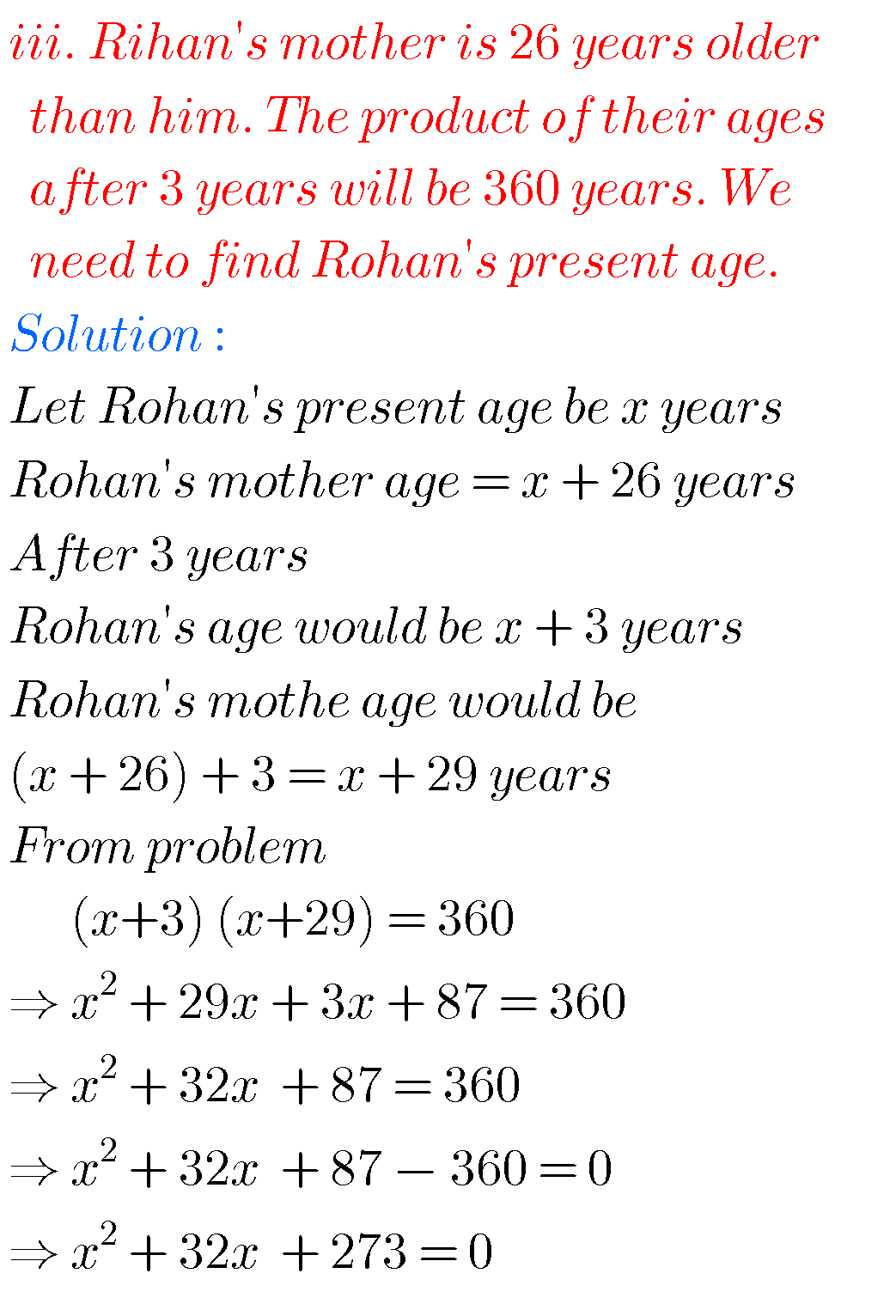## Solutions for Quadratic Equations ssc class 10 maths

Exercise 5.2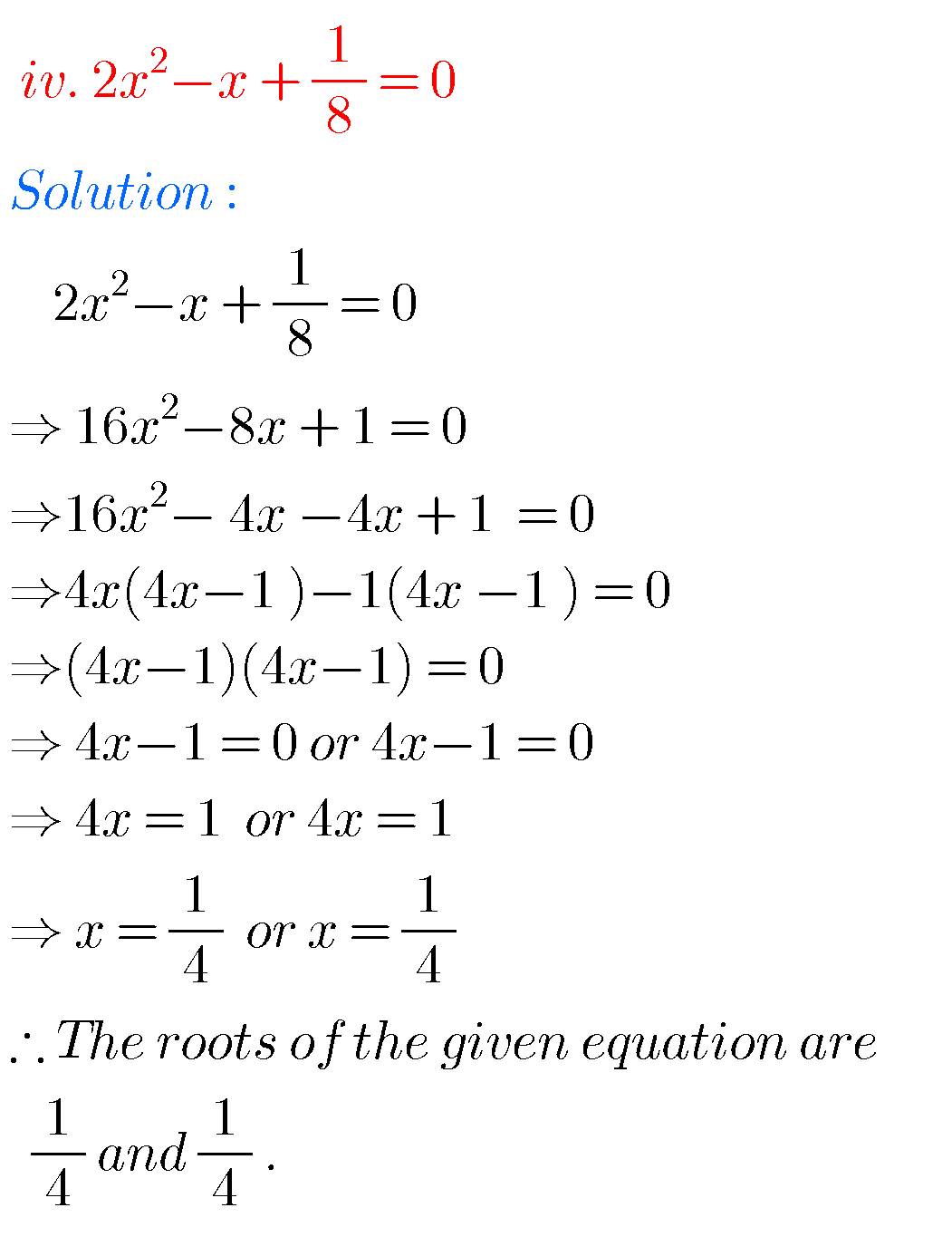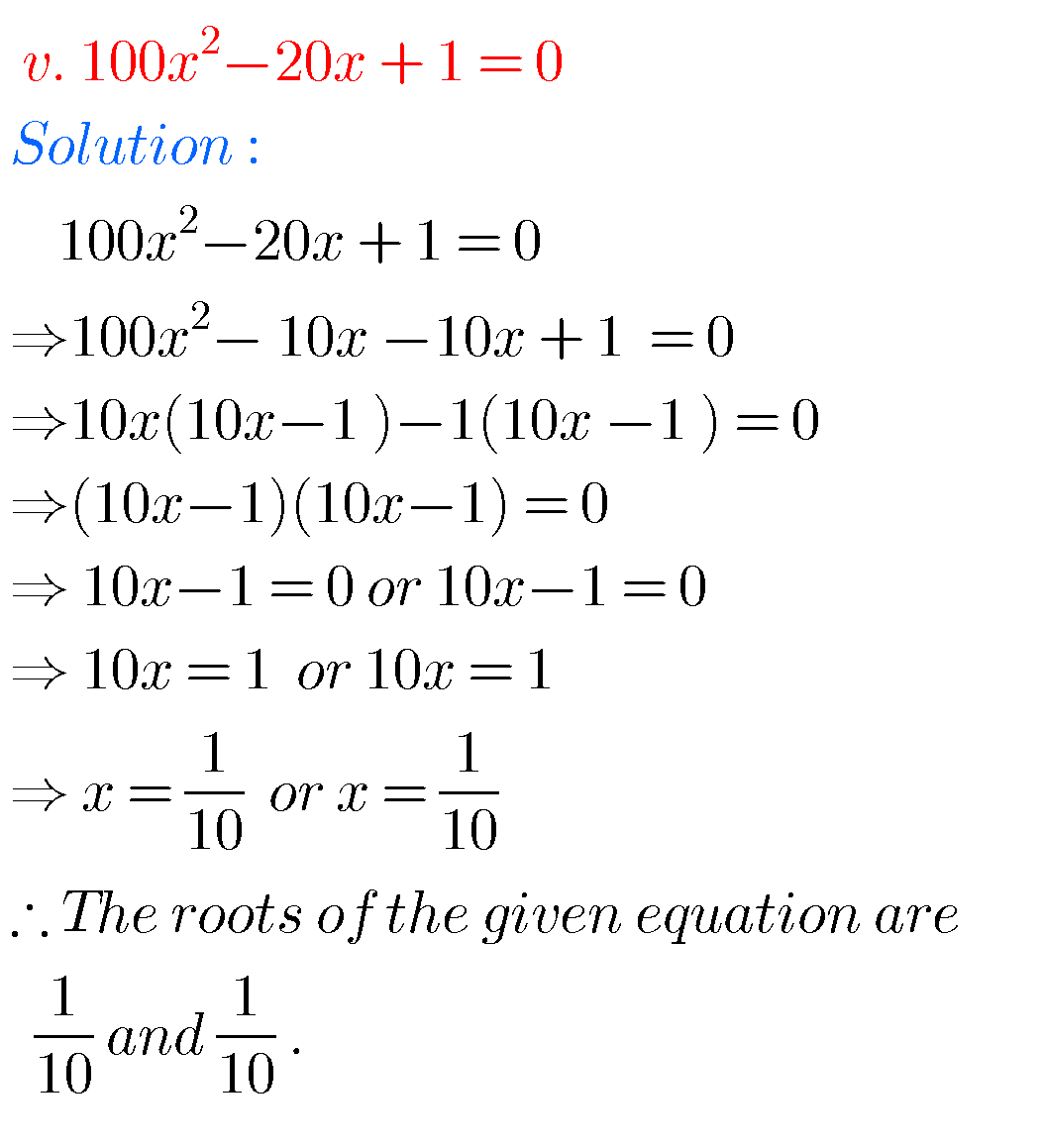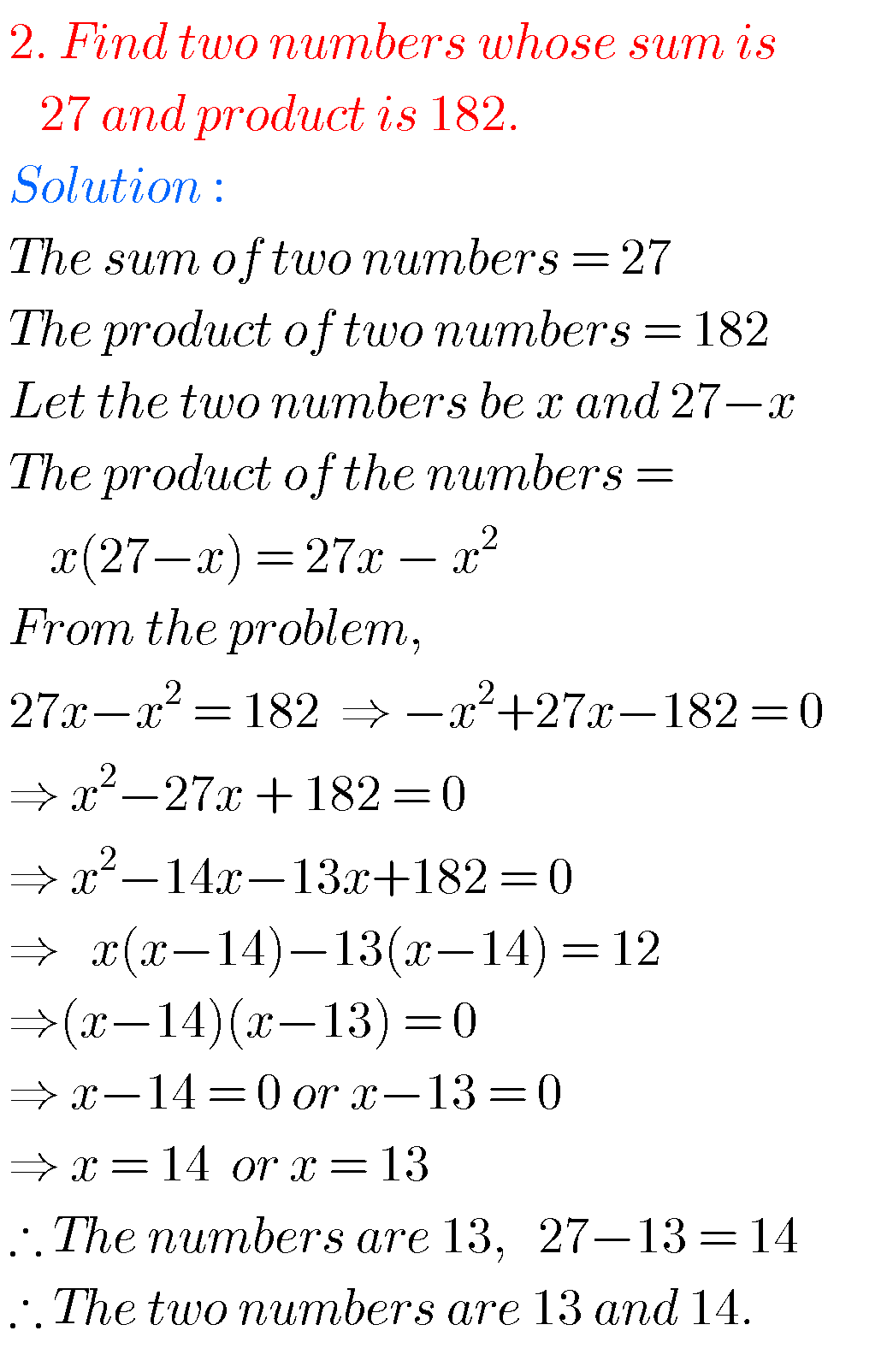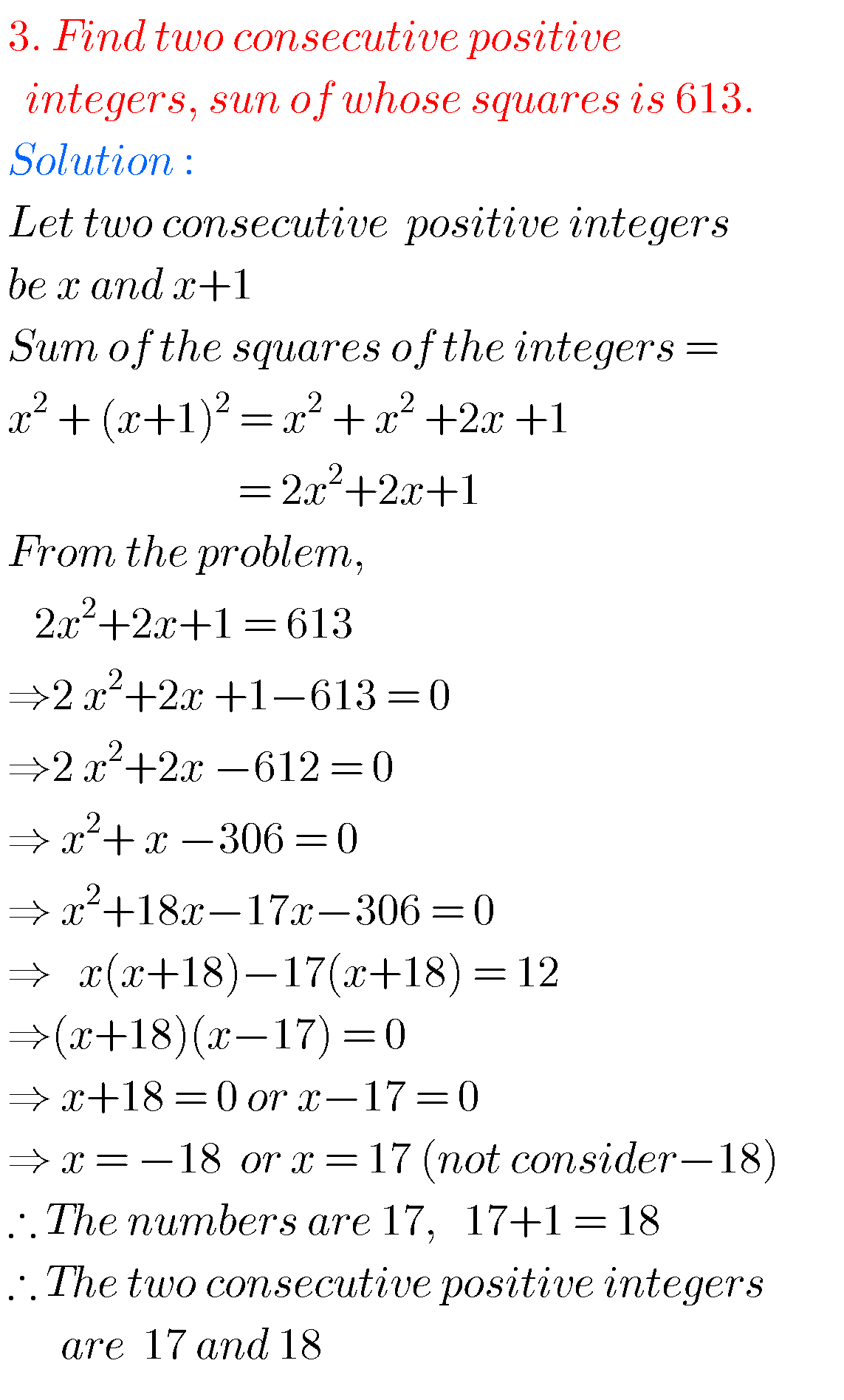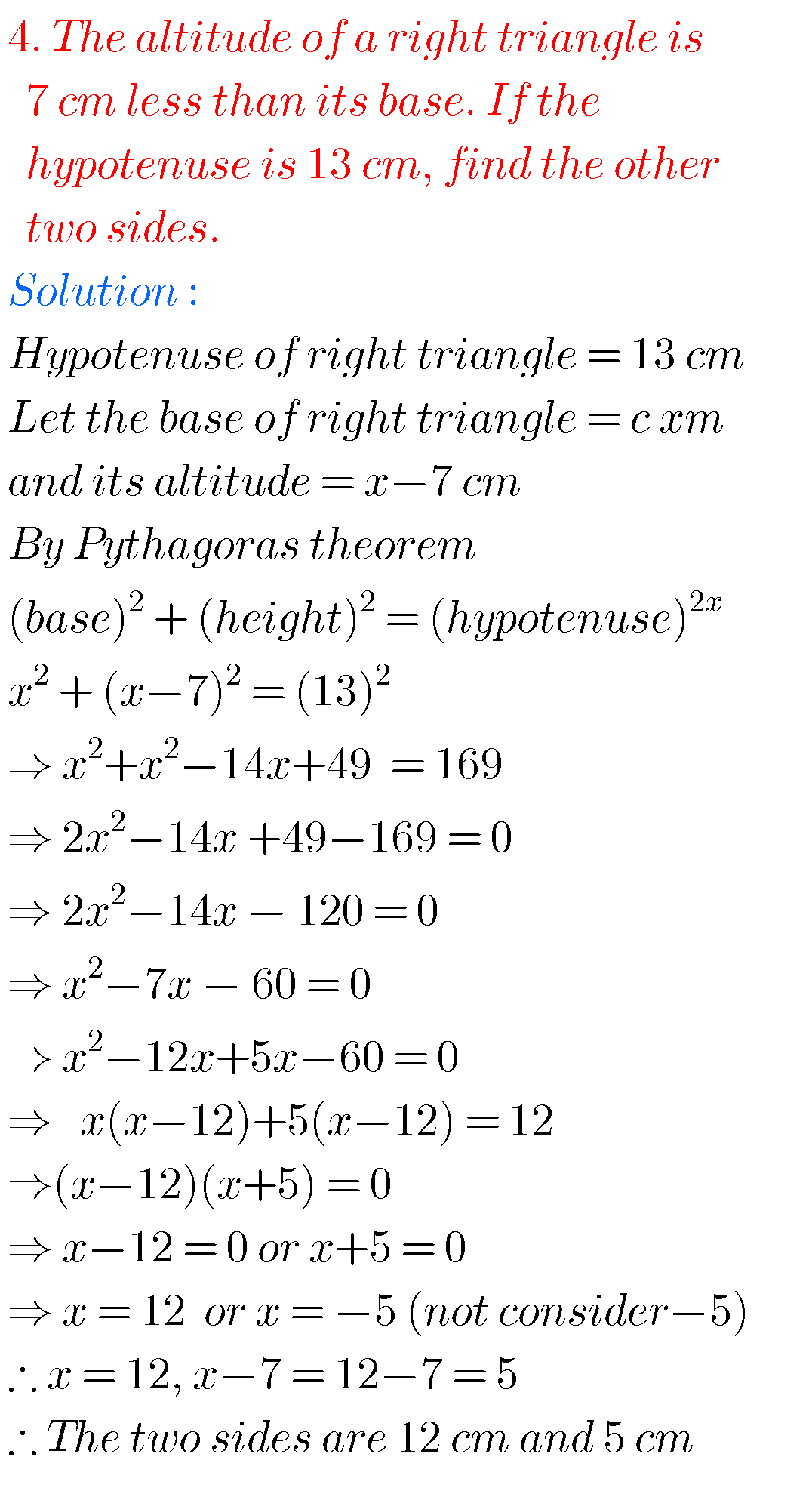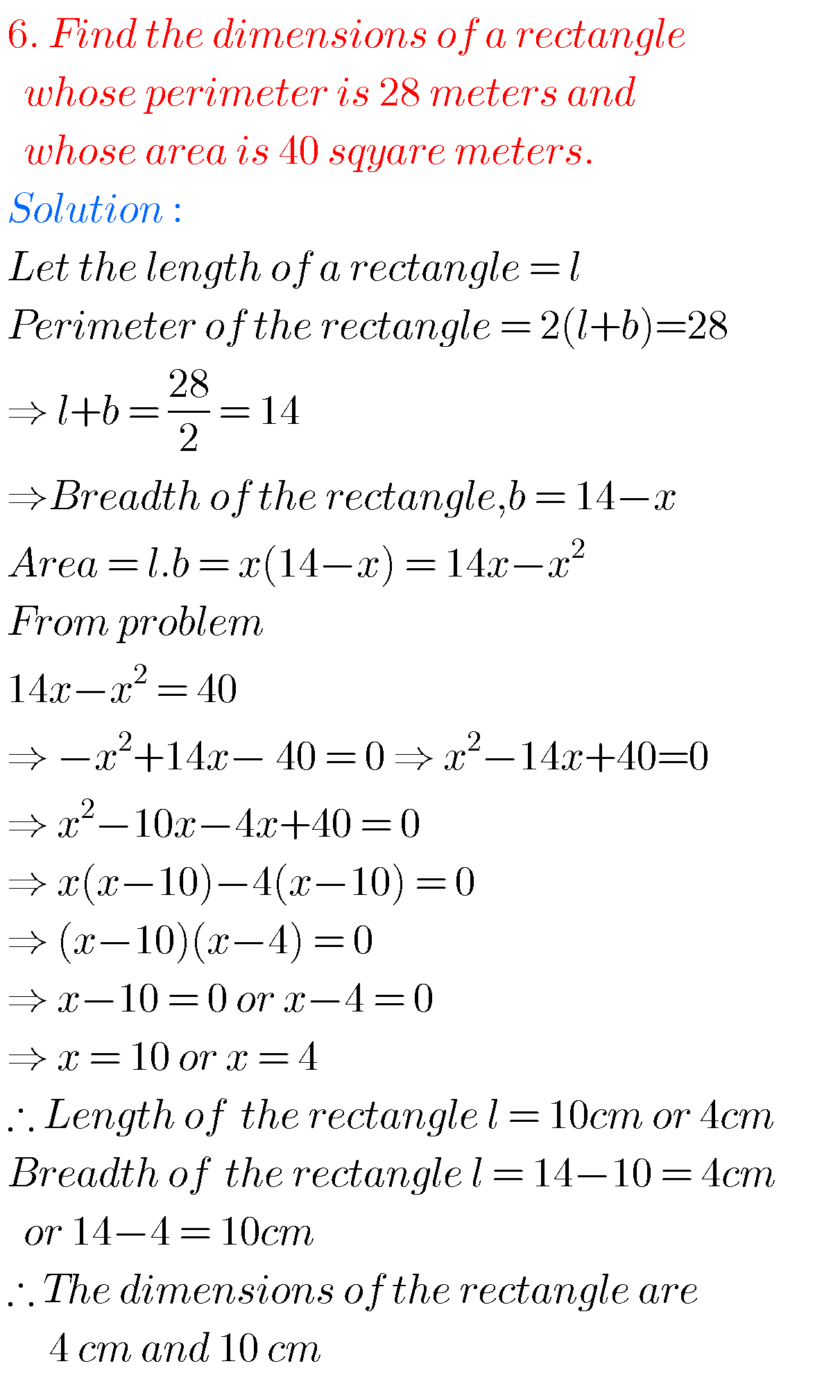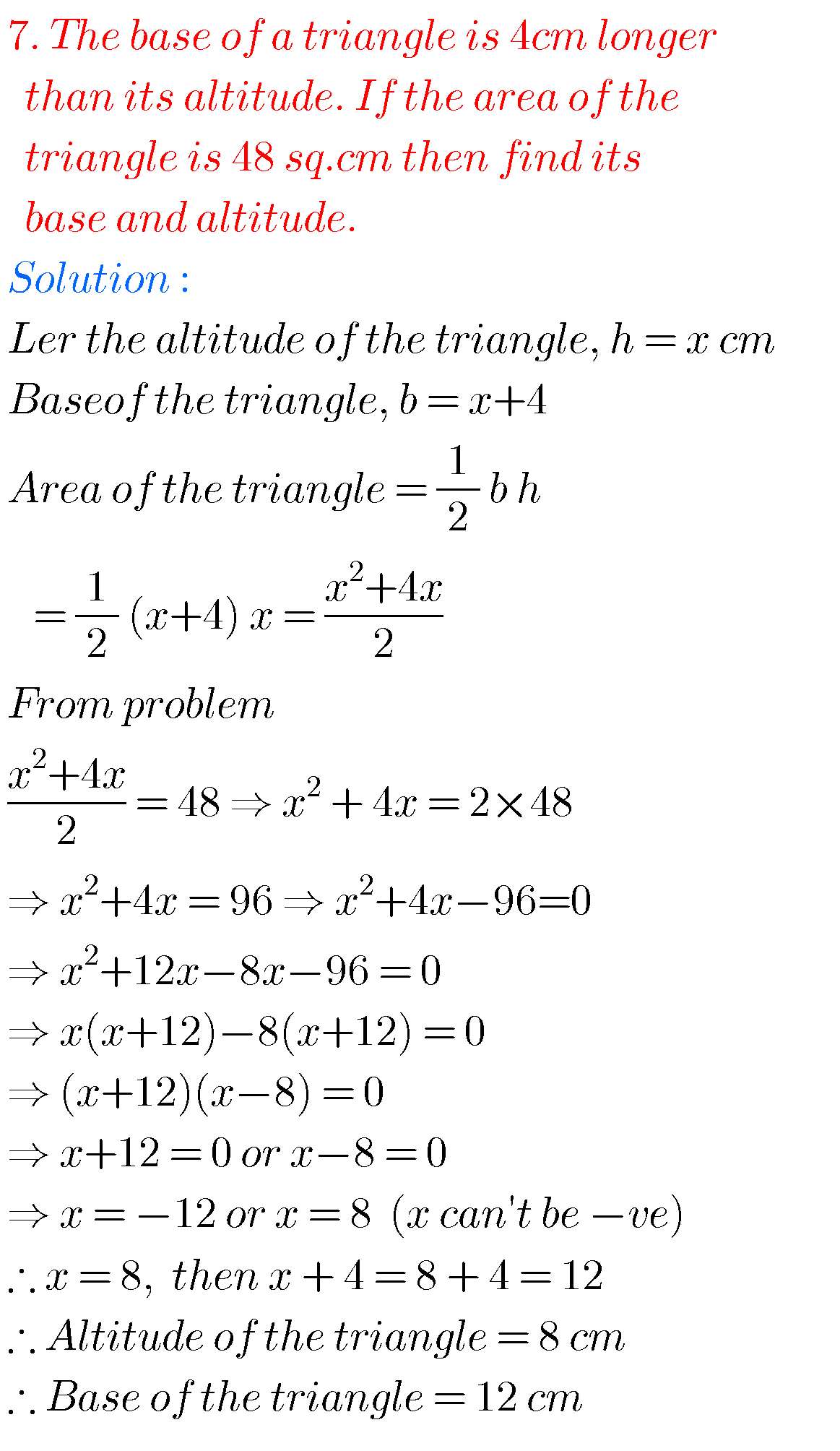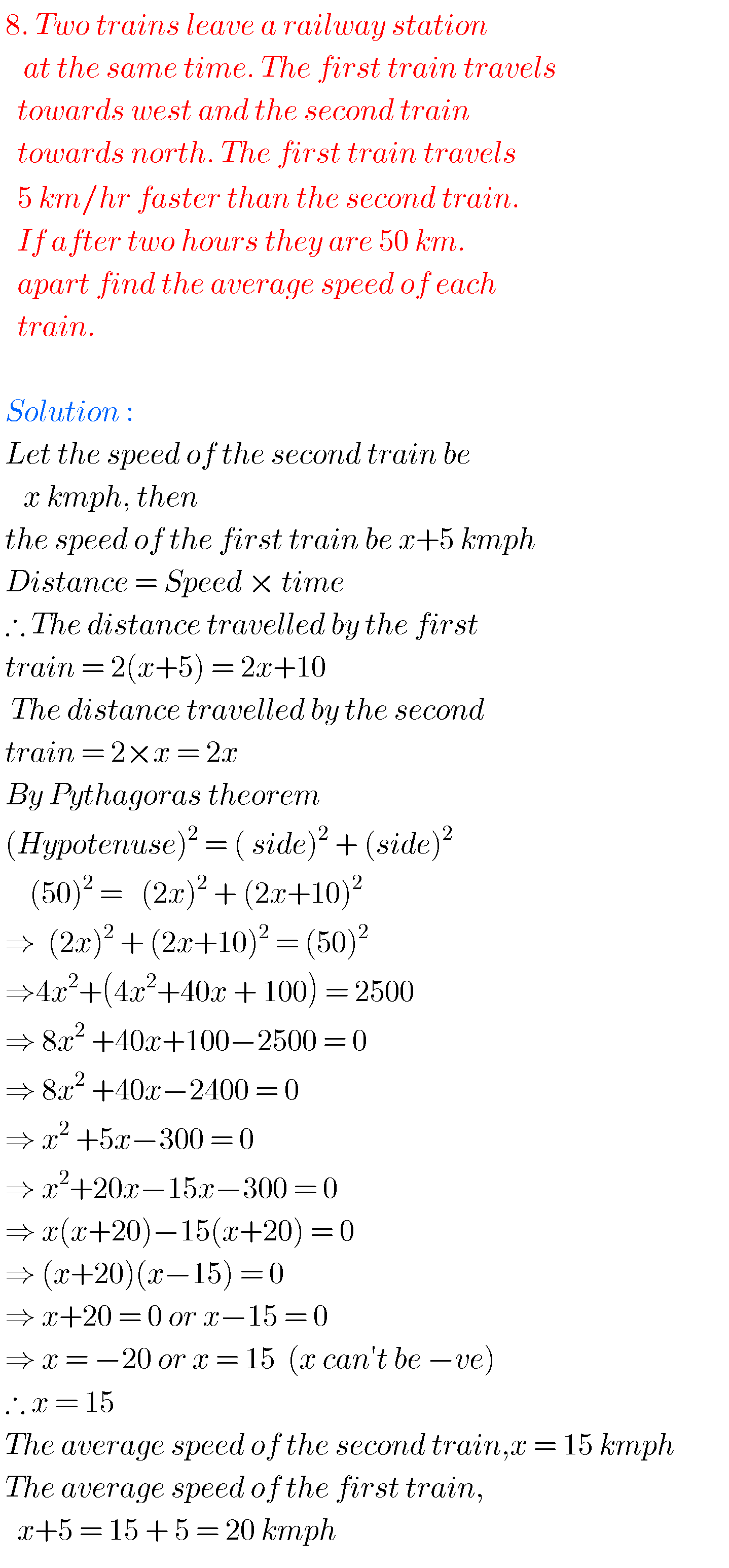## Maths solutions for Quadratic Equations class 10

Exercise 5.3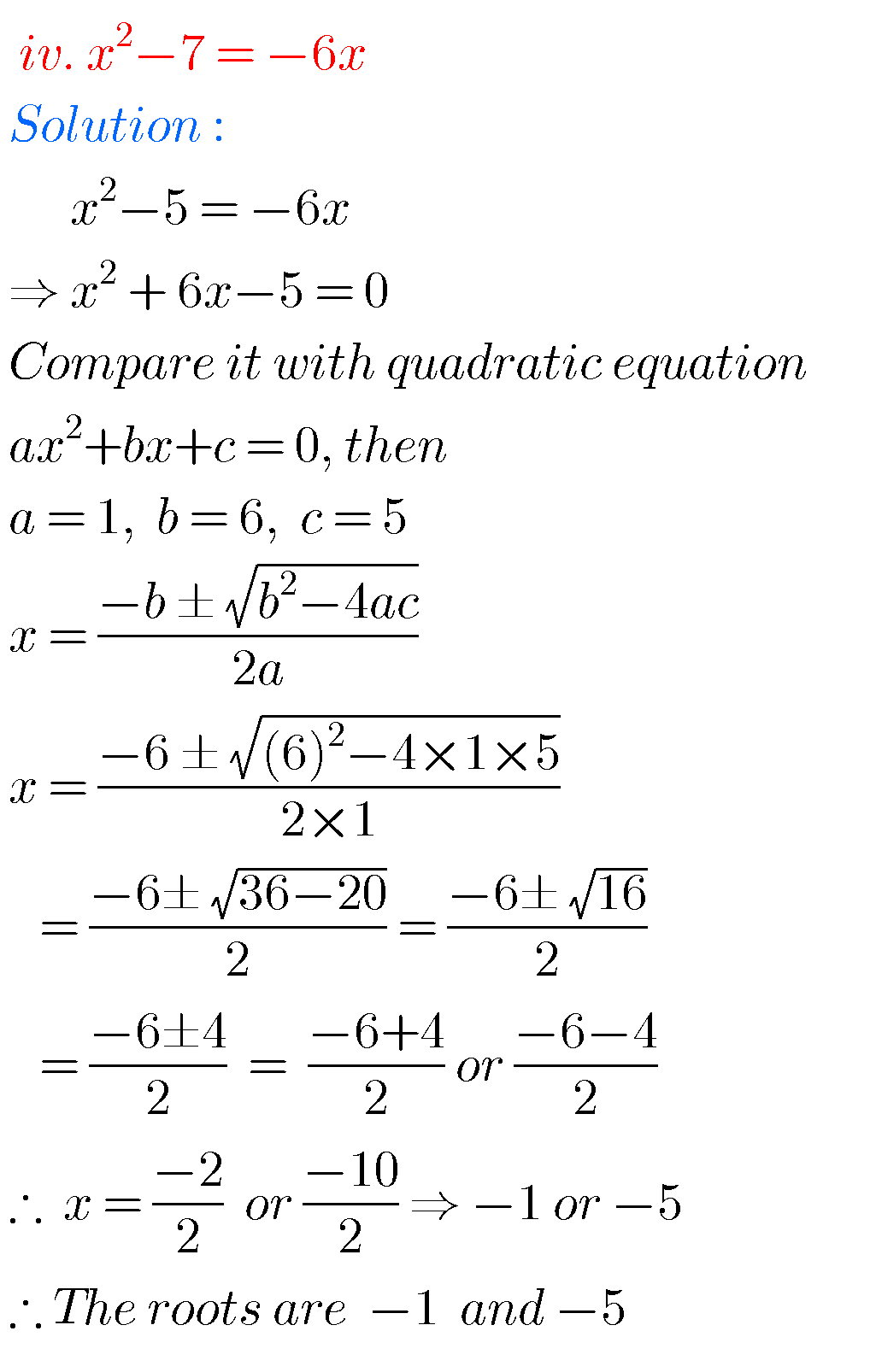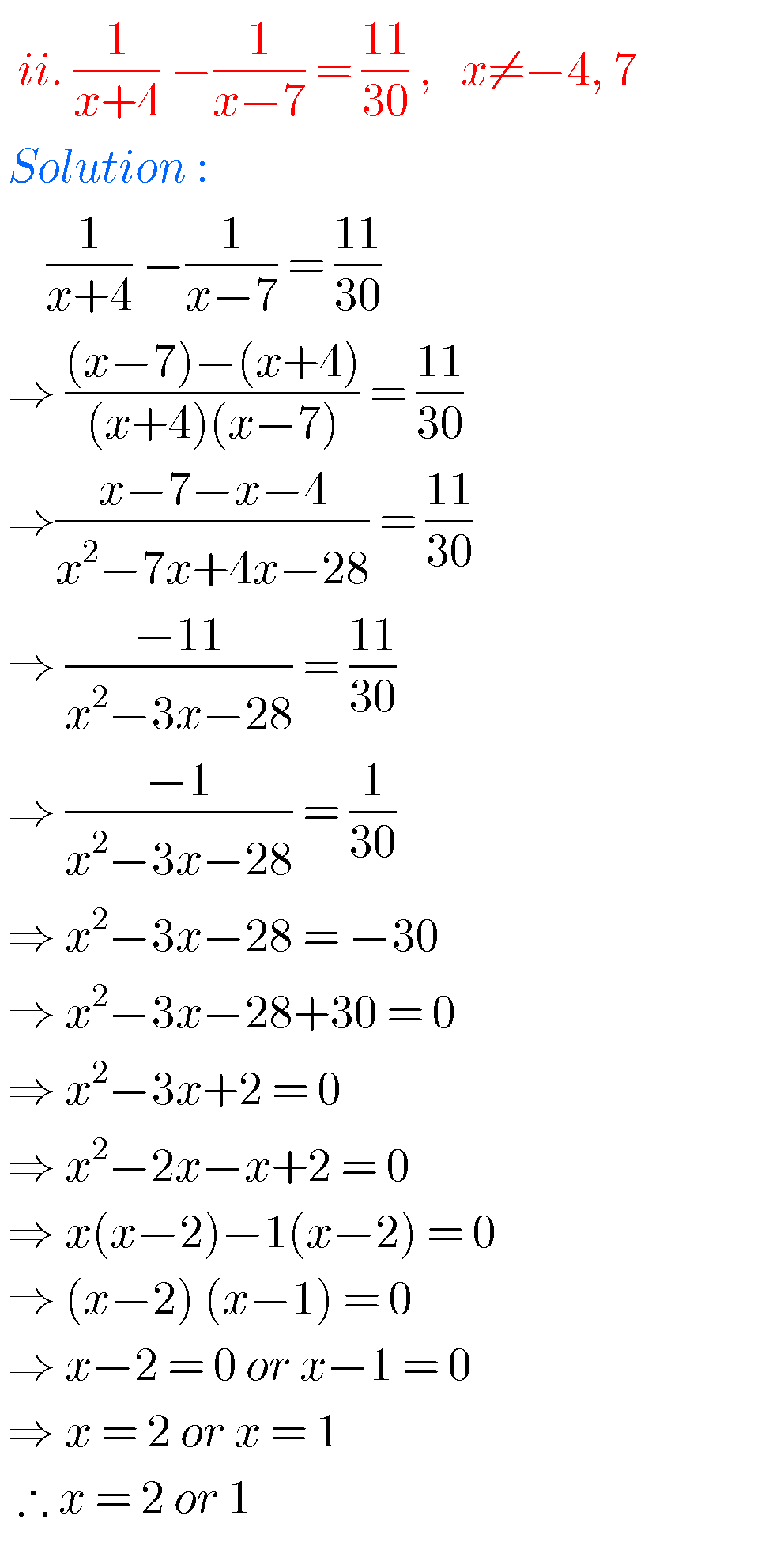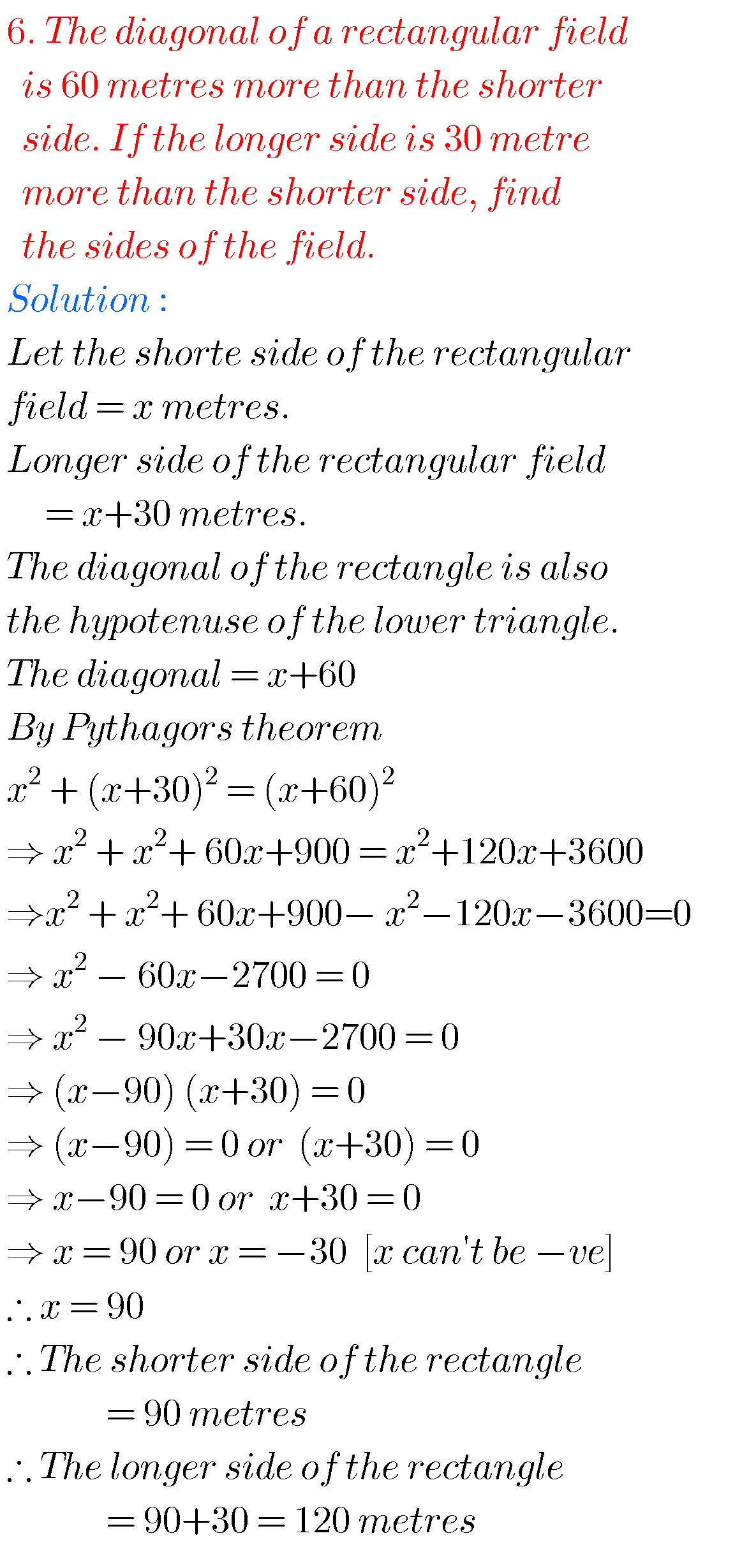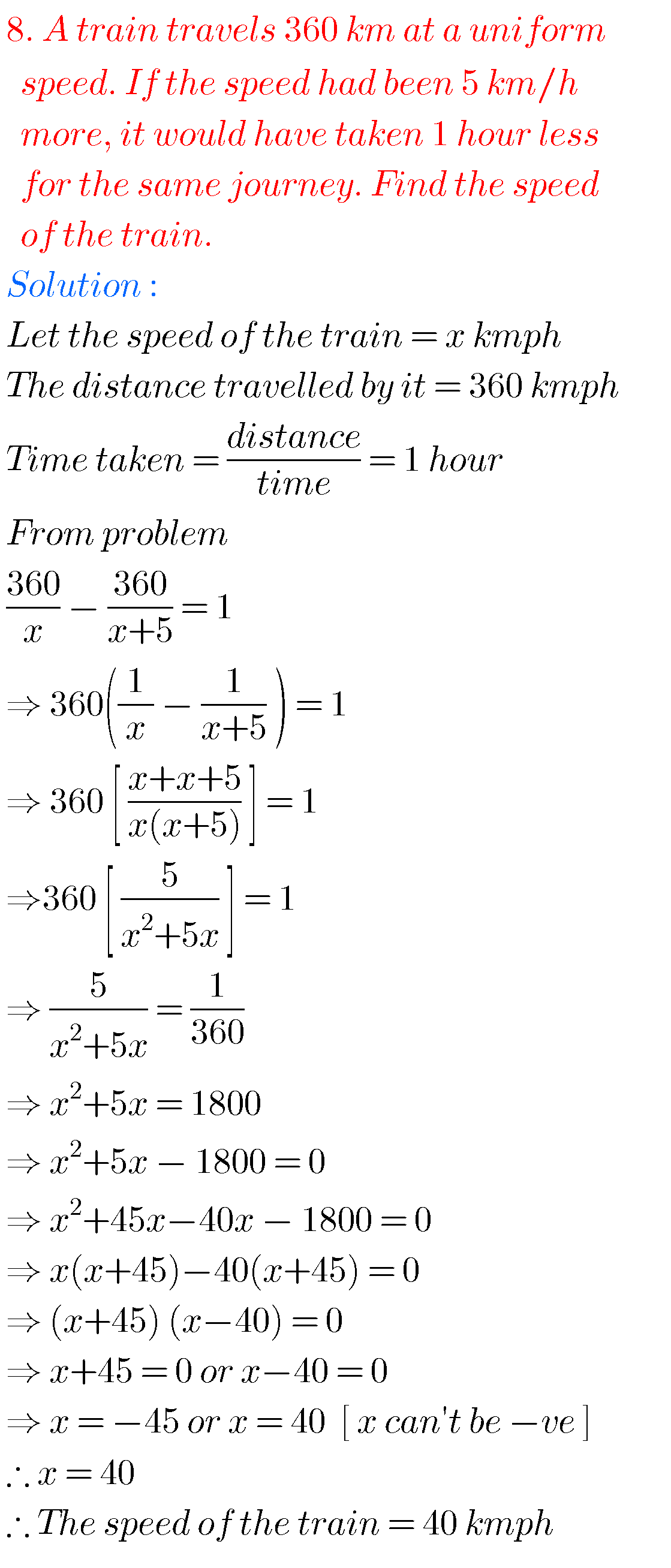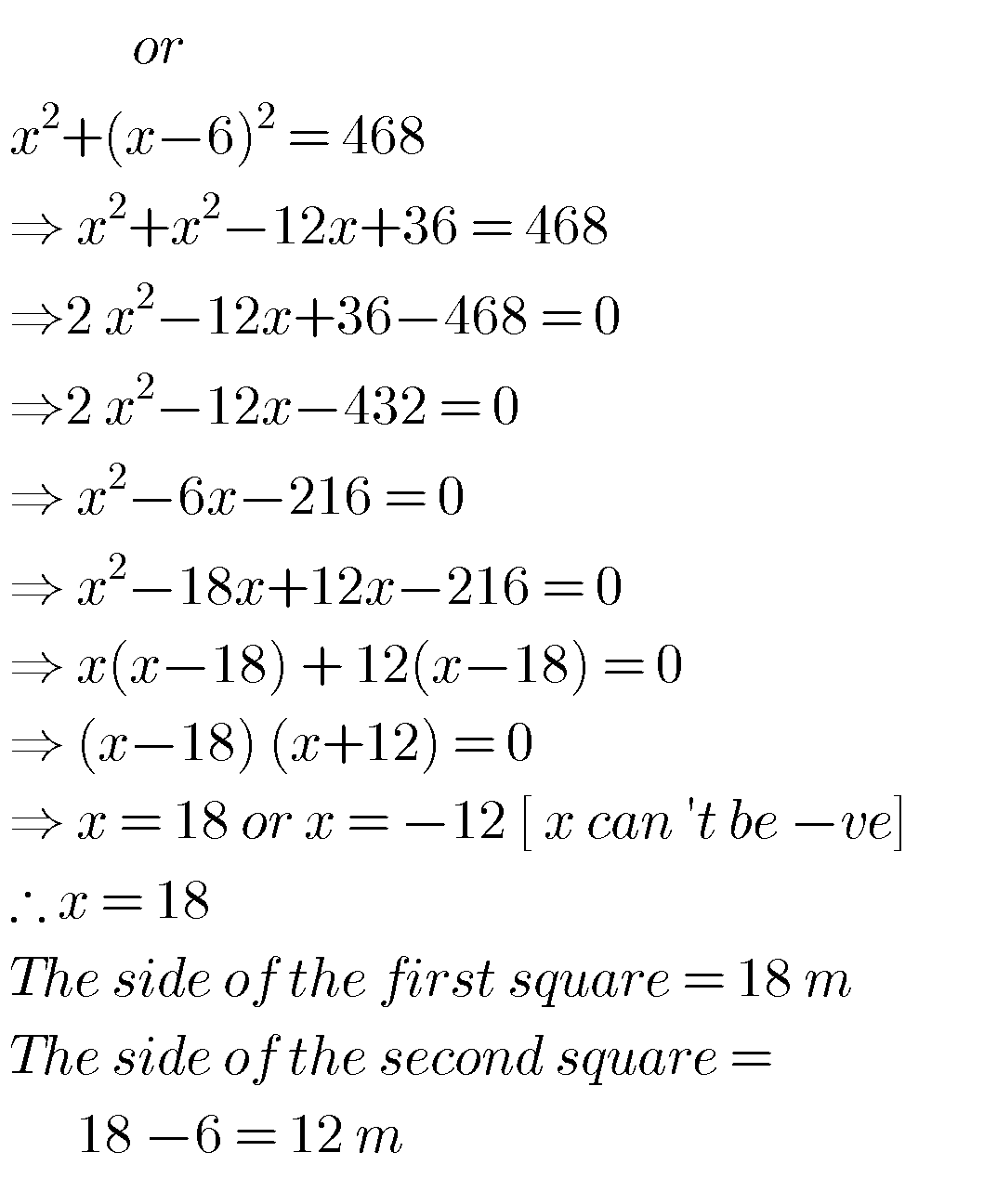### Tenth class Quadratic equations solutions ssc maths

Exercise 5.4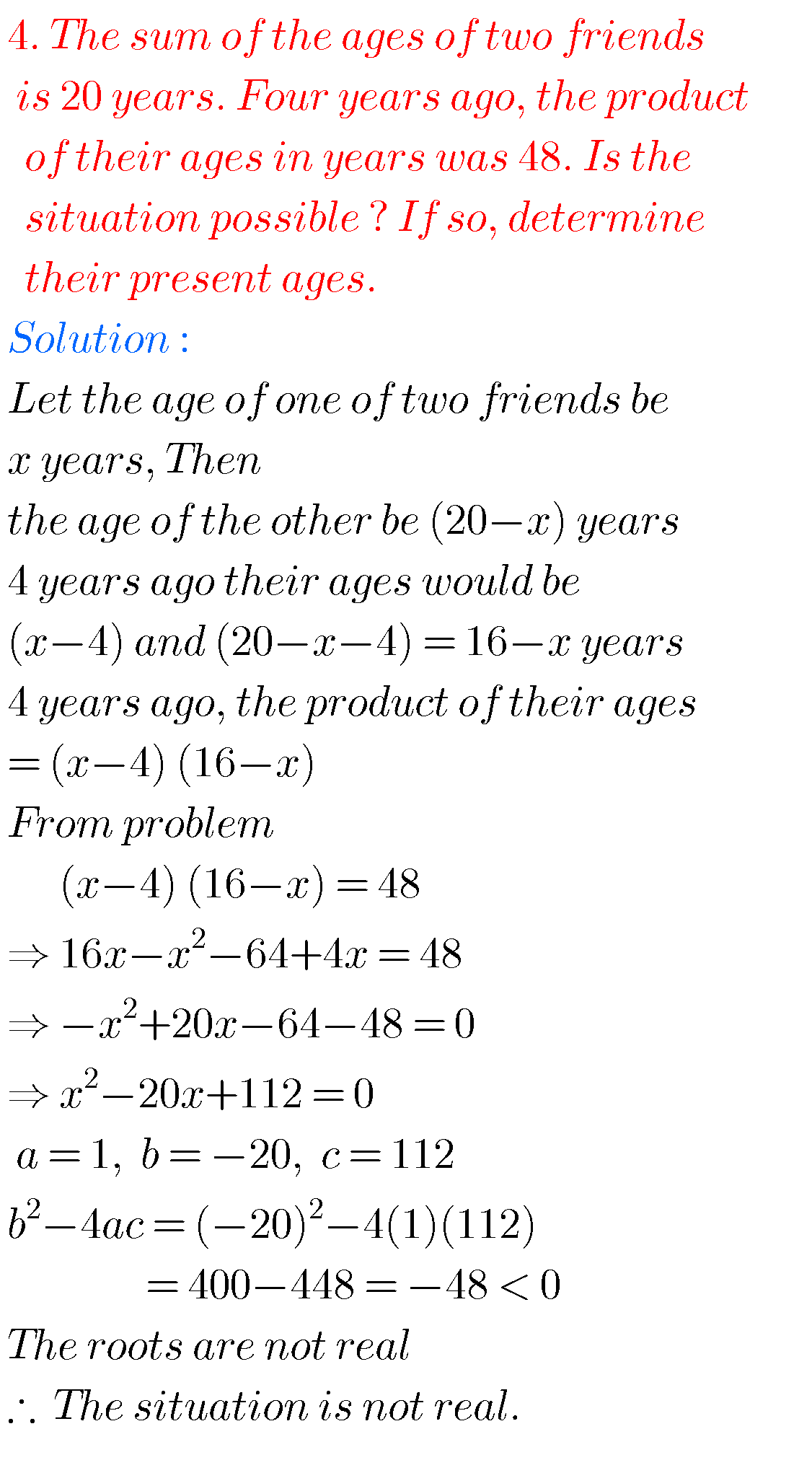Optional Exercise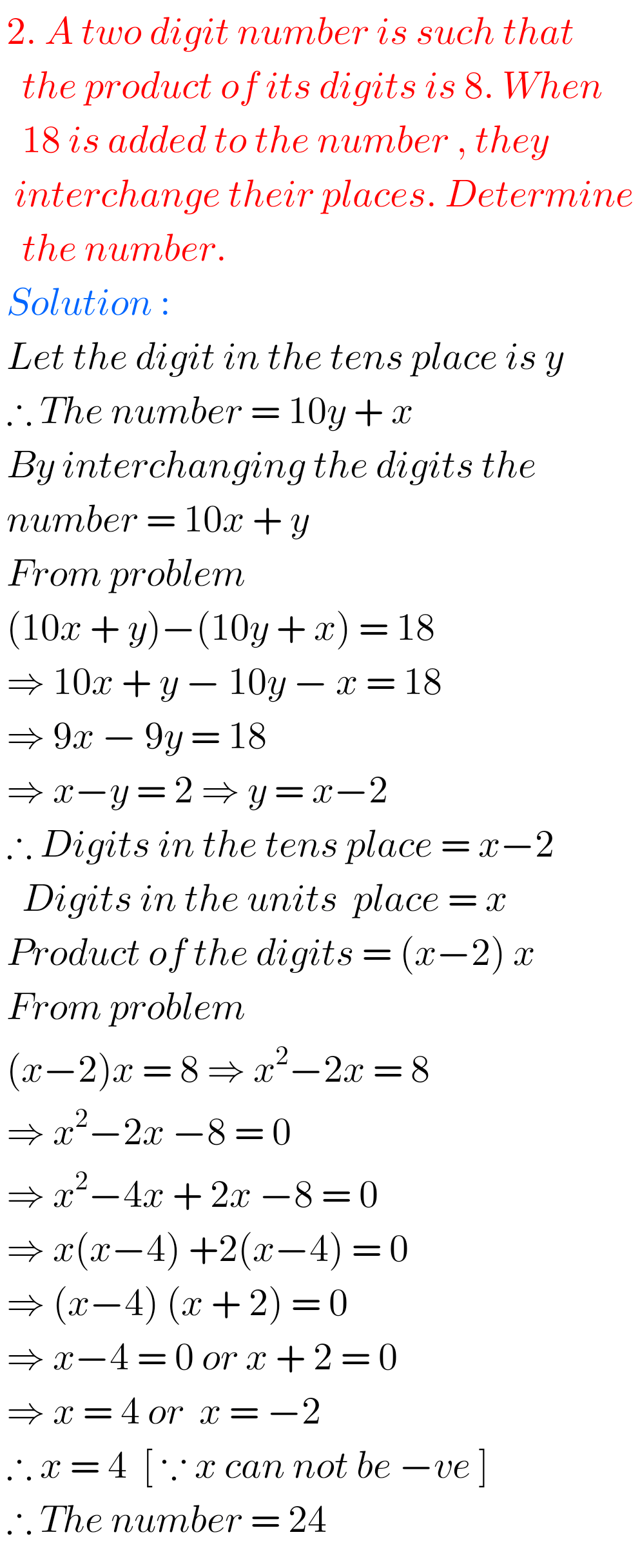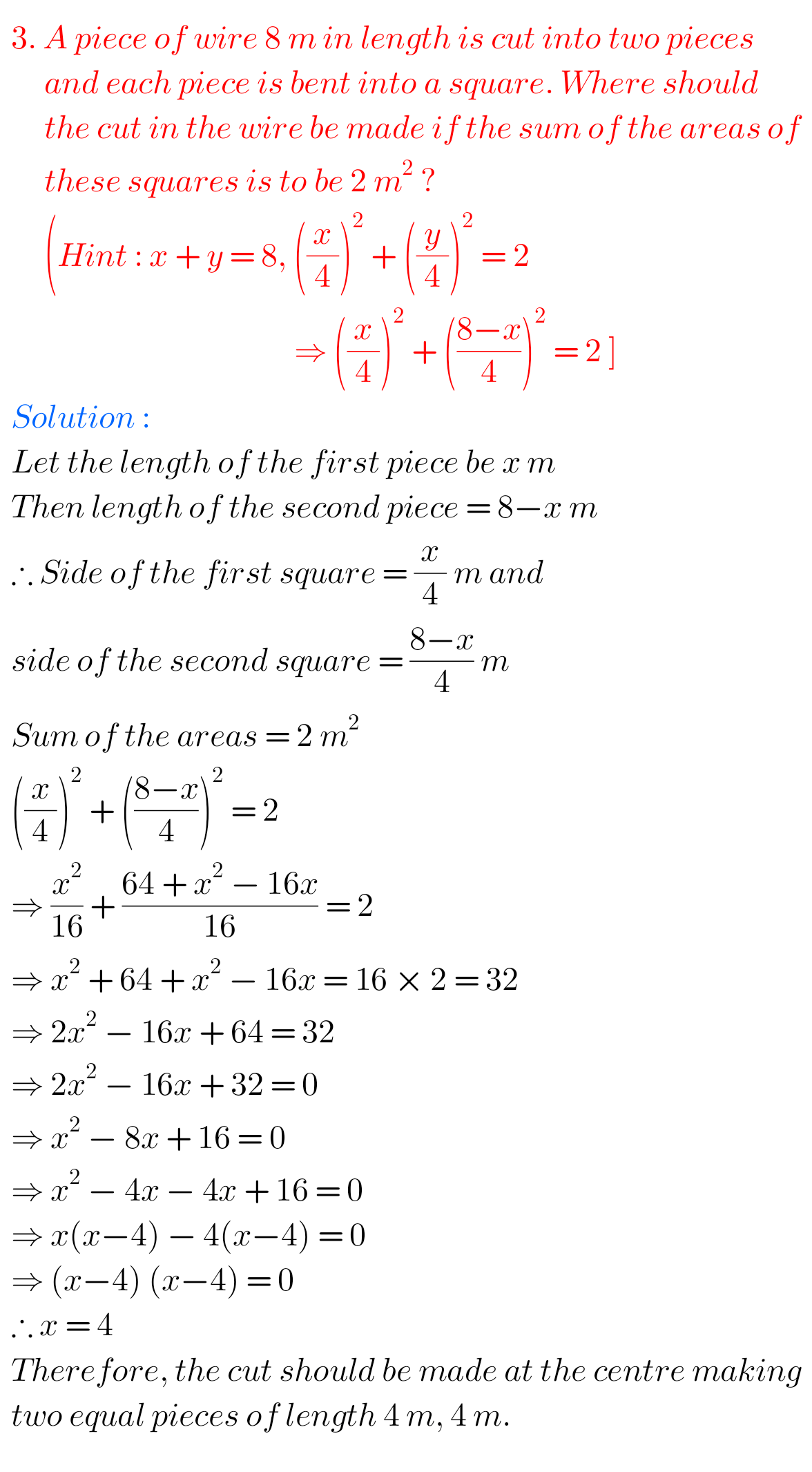M

Note :

Observe the solutions and try them in your own method.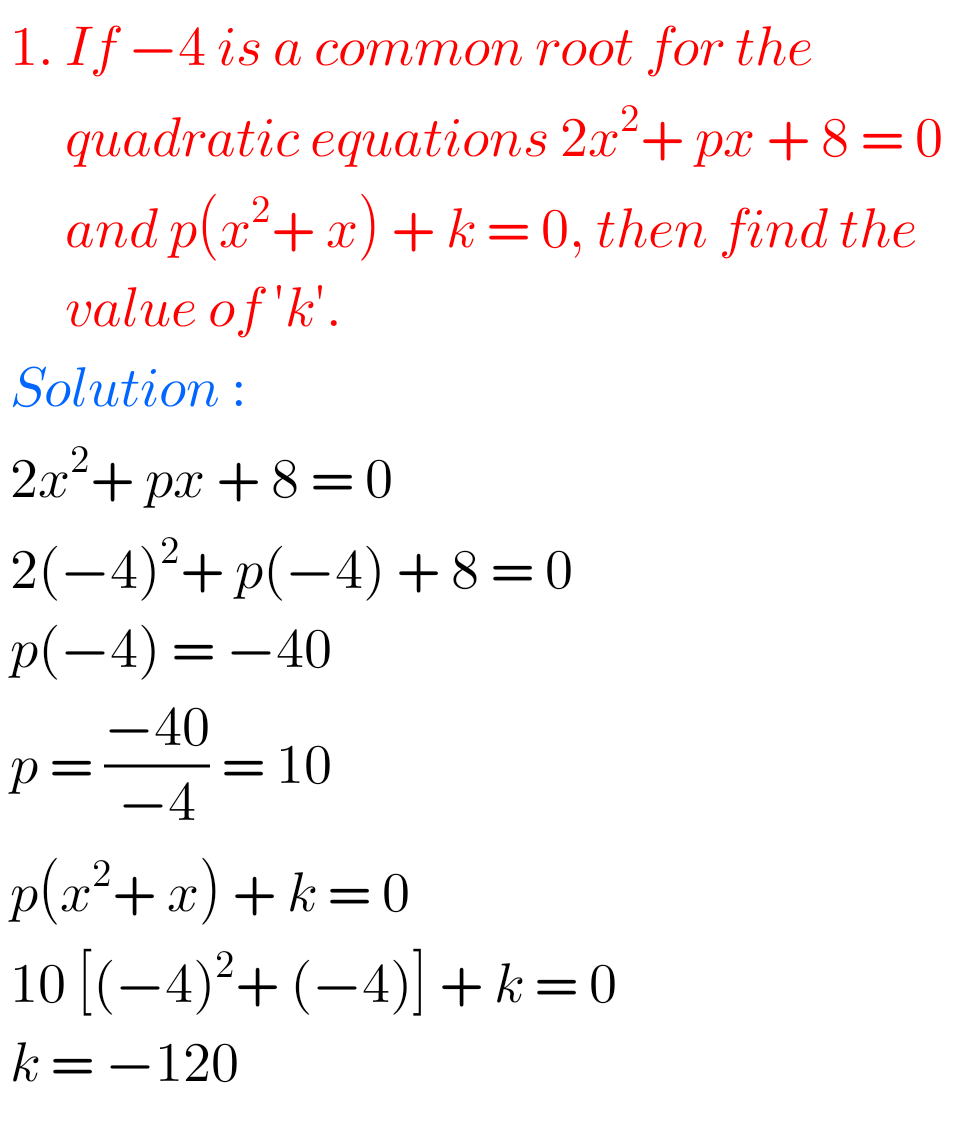Some solutions for different classes

Inter 1a exercise 6(a) solutions

Inter maths 1a exercise 6(b) solutions

class 10 maths polynomials solutions

Ncert maths class 8 exponents and powers

Ncert maths class 7 fractions and decimals

Ncert maths class 6 playing with numbers

Ncert solutions for maths class 7 rational numbers

Ncert solutions for maths class 6 knowing our numbers

Ncert solutions for maths class 6 integers

Ncert solutions for maths class 7 integers

### 7 thoughts on “SSC solutions for Maths Quadratic Equations class 10”

1.YELLA .SAMANVITHA REDDY

Thank u somuch for these answers these are very useful

2.3.Thank you thank you so much it is very very useful

4.Thank you some this is very very helpful

1.Thank you so much for the creator he/she is a very very good and this sums in this content are very useful

5.6.